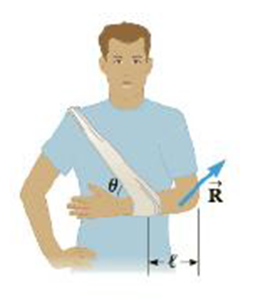Chapter 8, Problem 92AP

Chapter
Section
Textbook Problem

In an emergency situation, a person with a broken forearm ties a strap from his hand to clip on his shoulder as in Figure P8.92. His 1.60-kg forearm remains in a horizontal position and the strap makes an angle of θ = 50.0° with the horizontal. Assume the forearm is uniform, has a length of ℓ = 0.320 m, .assume the biceps muscle is relaxed, and ignore the mass and length of the hand. Find (a) the tension in the snap and (b) the components of the reaction force exerted by the humerus on the forearm.Figure P8.92

(a)

To determine
The magnitude of tension in the strap.

Explanation

Given Info:

Mass of the forearm is 1.60kg , the strap which makes angle with horizontal position is 50.0° , the distance from the elbow to the forearm is 0.320m .

Figure shows components of tension on the strap, components of reaction forces on the forearm, and weight of the forearm.

The arm is at rest. So, the equilibrium condition for the arm to be at rest is,

τ=0

Net torque acting on the arm is zero.

Consider the elbow as pivot and take torque about it.

The above expression can be written in terms of tension is given by

(Tsinθ)l(l2)mg=0

Rewrite the above expression in terms of T

T=(12)mgsinθ

Substitute 1

(b)

To determine
The magnitude of components of reaction forces.

Still sussing out bartleby?

Check out a sample textbook solution.

See a sample solution

The Solution to Your Study Problems

Bartleby provides explanations to thousands of textbook problems written by our experts, many with advanced degrees!

Get Started